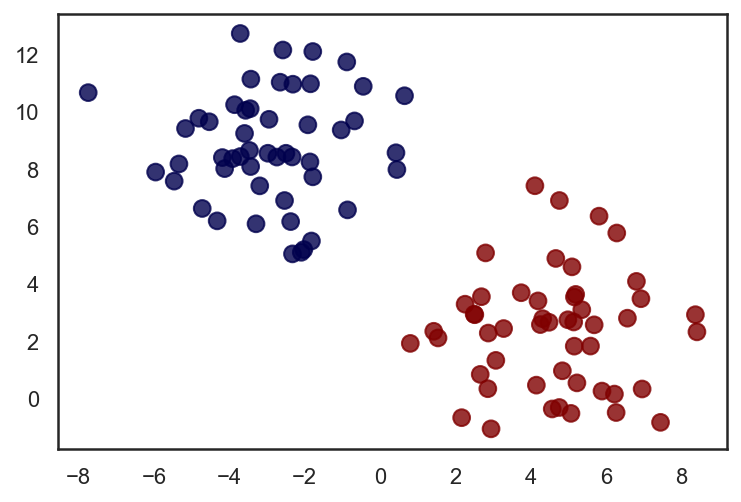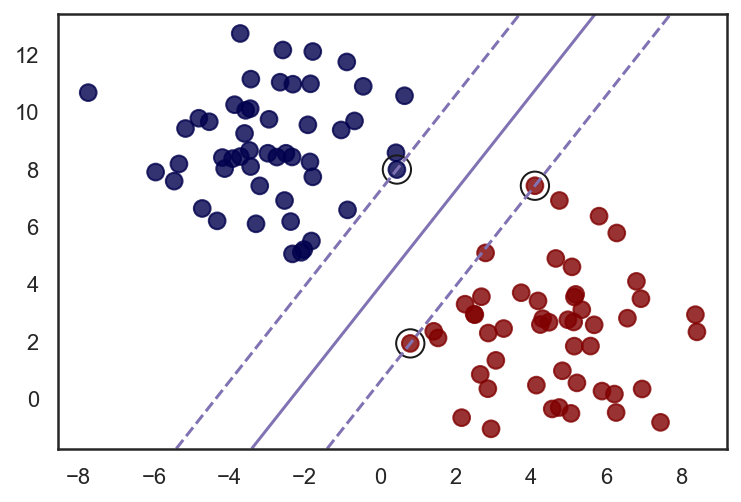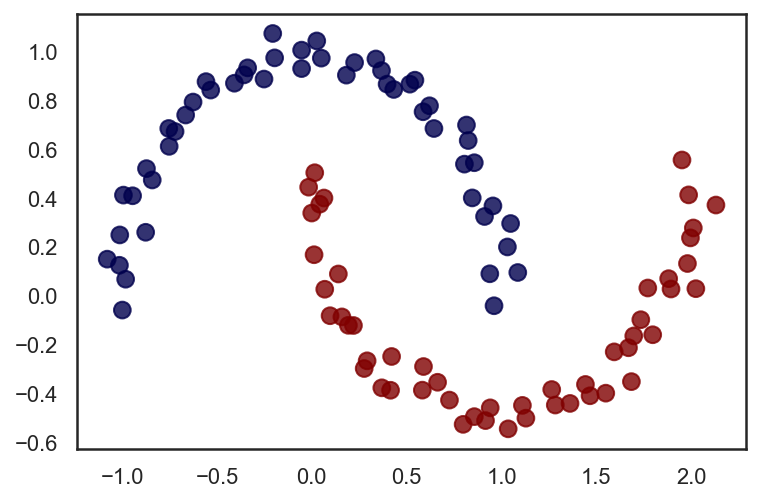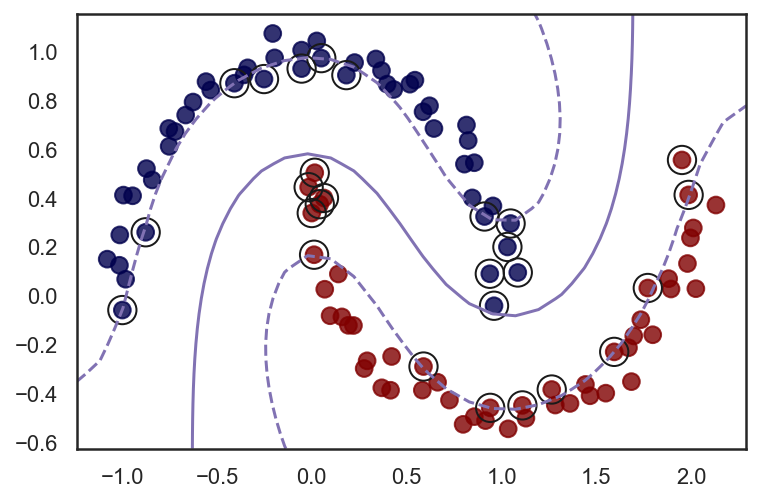# Support Vector Machine¶

Author: Olatomiwa Bifarin.
PhD Candidate Biochemistry and Molecular Biology
@ The University of Georgia

This is a draft copy, a work in progress

## Notebook¶

In :
# More sharp and legible graphics
%config InlineBackend.figure_format = 'retina'

import numpy as np
import pandas as pd
from matplotlib import pyplot as plt
from matplotlib import style
import sklearn
#For Seaborn plots
import seaborn as sns; sns.set(style='white')


## 1. Introduction¶

In support vector machine, the goal here is to fit a gutter to (two) linearly separable groups of samples. To achieve this goal, a margin is defined by the support vectors; and an optimal hyperplane somewhere in the middle (see figure below).Given a feature set $x$, the decision function (optimal hyperplane) is given below:

$$\large b + \mathbf{w}\mathbf{x}$$

Where b and $\mathbf{w}$ are the bias and weight values respectively. During training process, the optimal value for b and w is determined by solving a constrained optimization process defined as such:

$$\large \underset{w,b}{\text{Minimize}} \frac {1}{(w,b)}$$

$$\large \text{subj. to } y (b + \mathbf{w} . \mathbf{x}) \geq 1$$

The goal is to search for the optimal values of w and b that maximizes the margin (i.e. makes it as large as it could get), subject to the constraint that all training examples are classified correctly.

What has been described so far will be referred to as the hard margin SVM; hard because it is only applicable where the dataset is linearly separable (recall the constraint). What if they are not? What if the datasets aren’t linearly separable? The optimization problem as stated above will be unsolvable.

To make it solvable, a slack parameter will be included – for each training example – into the optimization calculations. The slack variables help to compensate for samples on the wrong side of the decision function during the training process (because of the absence of a perfect linear boundary). This is done by moving the samples to the right side.As such we can define our new optimization problem below:

$$\large \underset{w,b,\xi}{\text{Minimize}} \frac {1}{\gamma(w,b)} + C \sum \xi$$

$$\large \text{subj. to } y (b + \mathbf{w} . \mathbf{x}) \geq 1 - \xi$$$$\large \xi \geq 0$$

Our goal now is slightly edited: to make the margin as large as possible ($\frac {1}{\gamma(w,b)}$), and to make the slack parameter as small as possible ($C \sum \xi$); subject to the constraint that all training samples are correctly classified given the slack variables. The hyperparameter C controls underfitting/overfitting. And, friends, this, is the soft margin SVM

Before we proceed to other speaking points like kernel SVM, and how we will proceed with prediction (which should be damn obvious); let’s treat this optimization problem mildly, it should be fun.

## 2. Optimization and Prediction¶

Now, that we have abstracted out the optimization problem to be solved, let’s see if we can articulate it a little bit more. Okay. First, how do we make the margin as large as possible? We start by recognizing that the slope of the hyperplane will be $‖w‖$, the norm of the weights.And what good will this do for us? Plenty. I wouldn’t proof it here, but it turns out that if we divide $‖w‖$ by, say, 2; the margin increases by that factor. So, to maximize the margin, we minimize the weights.

$$\large \underset{w,b,\xi}{\text{Minimize}} \frac {1}{2}‖w‖^2 + C \sum \xi$$

$$\large \text{subj. to } y (b + \mathbf{w} . \mathbf{x}) \geq 1 - \xi$$$$\large \xi \geq 0$$

Finally, after training, prediction proceeds follows:

$$\large b + \mathbf{w}\mathbf{x} \leq -1, c=0$$$$\large b + \mathbf{w}\mathbf{x} \geq -1, c=0$$

## 3. Kernelized SVM¶

The notes above had highlighted how SVM can be used in linear classification. However, SVM is more versatile as it is can be used for non-linear classification, and even for regression. In this section, we will focus on non-linear SVM classifications. If we do have a classification problem where samples are not linearly separable (see figure below), the next thing to try will be a kernelized SVM.In the figure above the data in a 1-D space isn’t linearly separable, however, mapping to a 2D space renders it linearly separable. For a non-linear SVM classification, the features are mapped from the native feature space to a higher dimensional space that permits linear separability. In Kernelized SVM, we use what is called a kernel trick. The idea of a kernel trick is as follows: to map a set of samples to a higher dimensional space is computationally expensive, as such, we ask – can we get the results of a transformation without going through the computational expense it affords? It turns out we can. Here is an example:

$$\large \phi(p) = \phi \begin{bmatrix} p_{1} \\ p_{2} \end{bmatrix} = \begin{bmatrix} p_{1}^{2} \\ \sqrt{2} p_1p_2 \\ p_{2}^{2} \end{bmatrix}$$

Where $\phi(.)$ is a second degree polynomial transformation. Notice the mapping from a 2D space to a 3D space.

Below we see the dot product of two of such transformation:

$$\large \phi(p).\phi(q) = \begin{bmatrix} p_{1}^{2} \\ \sqrt{2} p_1p_2 \\ p_{2}^{2} \end{bmatrix} \begin{bmatrix} q_{1}^{2} \\ \sqrt{2} q_1q_2 \\ q_{2}^{2} \end{bmatrix} = p_{1}^{2}q_{1}^{2} + 2p_{1}q_{1}p_{2}q_{2} + p_{2}^{2}q_{2}^{2}$$
$$\large = {(p_{1}q_{1} + p_{2}q_{2})}^2 = \begin{bmatrix} \begin{bmatrix} p_{1} \\ p_{2} \end{bmatrix} . \begin{bmatrix} q_{1} \\ q_{2} \end{bmatrix} \end{bmatrix}^2= (\mathbf{p}.\mathbf{q})^2$$

Hopefully now you can observe the miracle : we can deduce the dot product of two 2nd degree polynomial transformation by taking the square of the dot product. That is, we can transform the dataset without computing it. Mathematically having our cake and eating it.

Examples of kernelized SVM include the following:

$$\large \text{Polynomial kernel: } k(p,q) = (\mathbf{p}.\mathbf{q} + r)^d$$$$\large \text{Gaussian radial basis function: } k(p,q) = \text{exp}(-\gamma ||\mathbf{p}-\mathbf{q}||^2 + C)$$$$\large \text{Sigmoid kernel: } k(p,q) = \text{tanh}(\gamma \mathbf{p}^T \mathbf{q} + r )$$

Where $C$ = cost, $r$ = coefficient, and $d$ = degree

Enough of maths, let's try our hands on codes.

## 4. (Some) Implementation¶

### 4a. Linear SVM¶

#### Generate a linearly separable dataset¶

In :
from sklearn.datasets import make_blobs
X, y = make_blobs(n_samples=100, centers=2,
n_features=2, cluster_std=2.0,
random_state=42)
plt.scatter(X[:, 0],
X[:, 1],
alpha=0.8,
c=y,
s=70,
cmap='seismic'); #'seismic','twilight'#### Linear SVM model¶

In :
from sklearn.svm import SVC
# fit the model and get the separating hyperplane
clf = SVC(kernel='linear', C=1.0)
clf.fit(X, y)

Out:
SVC(kernel='linear')

#### Function for decision function figure¶

In :
def plot_svm (X, classifier):
'''
X: dataset (numpy array)
Y: classfier (Here, SVM model)

Output: Figure.
'''

plt.scatter(X[:, 0], X[:, 1], alpha=0.8, c=y, s=70, cmap='seismic'); #'seismic','twilight'

# plot the decision function
ax = plt.gca()
xlim = ax.get_xlim()
ylim = ax.get_ylim()

# create grid to evaluate model
xx = np.linspace(xlim, xlim, 30)
yy = np.linspace(ylim, ylim, 30)
YY, XX = np.meshgrid(yy, xx)
xy = np.vstack([XX.ravel(), YY.ravel()]).T
Z = classifier.decision_function(xy).reshape(XX.shape)

# plot decision boundary and margins
ax.contour(XX, YY, Z, colors='m', levels=[-1, 0, 1], alpha=1.0,
linestyles=['--', '-', '--'])

# plot support vectors
ax.scatter(classifier.support_vectors_[:, 0], classifier.support_vectors_[:, 1], s=200,
linewidth=1, facecolors='none', edgecolors='k')



#### Support vectors, margin, and hyperplane¶

In :
plot_svm(X, clf)
plt.show()### 4b. Non-linear SVM¶

#### Generate a dataset immune to linear separation¶

In :
from sklearn.datasets import make_moons
X, y = make_moons(n_samples=100, noise=0.05, random_state=42)
plt.scatter(X[:, 0],
X[:, 1],
alpha=0.8,
c=y,
s=70,
cmap='seismic'); #'seismic','twilight'#### RBF SVM model¶

In :
clf2 = SVC(kernel='rbf', C=1.0)
clf2.fit(X, y)

Out:
SVC()

#### Non-Linear SVM: Support vectors, margin, and hyperplane¶

In :
plot_svm(X, clf2)
plt.show()## References and Resources¶

• SVM, Wikipedia
• Jake VanderPlas, Python Data Science Handbook, Chapter 5: Machine Learning
• Hal Daumé III, A Course in Machine Learning, Chapter 7: Linear Models
• Aurelien Geron, Hands-On Machine Learning with Scikit-Learn & TensorFlow, Chapter 5: SVM
In [ ]: